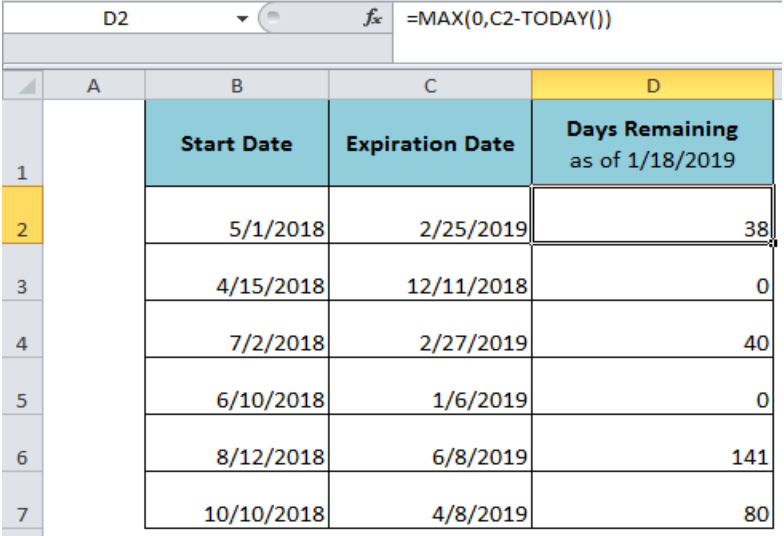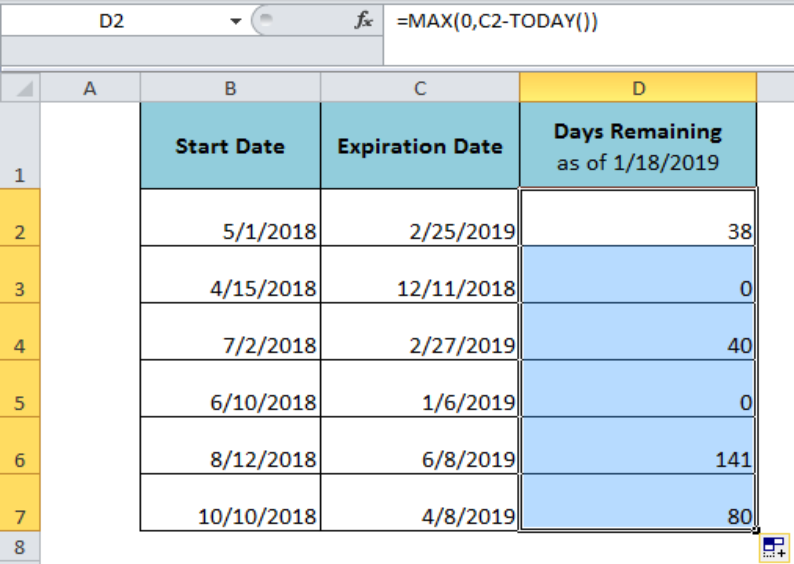Get instant live expert help with Excel or Google Sheets“My Excelchat expert helped me in less than 20 minutes, saving me what would have been 5 hours of work!”

#### Post your problem and you’ll get expert help in seconds.

Your message must be at least 40 characters
Our professional experts are available now. Your privacy is guaranteed.

# Calculate days remaining

When we have a targeted deadline to meet or an expiration date is given then we may need to calculate the days remaining until deadline date or expiration date from today. In some cases, we have two dates and we need to calculate the days remaining between these two dates.Figure 1. How to Calculate Days Remaining

## Calculate Days Remaining Until Expiration from Today

In case of an activity deadline date or a product expiry date, we need to calculate the days remaining until expiration or deadline date from today. In Excel, we can accomplish this task by subtracting Today’s date from expiration or deadline date, =Expiration Date – Today’s Date. But in case, the expiration date has passed before Today’s date then this formula will return the negative value. Therefore, the following amendment is needed to ignore the negative value and show 0 instead, such as;

`=MAX(0, Exp_date - Today_date)`

The MAX function will tackle the negative value and return 0 instead. In our example, we will insert this formula in cell D2 and copy down or drag the fill handle to get the days remaining in other calculations.

`=MAX(0,C2-TODAY())`Figure 2. Calculating Days Remaining as of Today

## Calculate Days Remaining Between Two Dates

If we have two dates given as start date and end date, and we need to calculate the days remaining between two dates, then we will simply subtract the start date from end date to get the difference in days, =End Date – Start Date. In our example, we will insert the following formula in cell D2 and drag down the fill handle or copy the formula to get the remaining calculations in other cells;

`=C2-B2`Figure 3. Calculating Days Remaining Between Two Dates

## Instant Connection to an Expert through our Excelchat Service

Most of the time, the problem you will need to solve will be more complex than a simple application of a formula or function. If you want to save hours of research and frustration, try our live Excelchat service! Our Excel Experts are available 24/7 to answer any Excel question you may have. We guarantee a connection within 30 seconds and a customized solution within 20 minutes.

### Did this post not answer your question? Get a solution from connecting with the expert.Another blog reader asked this question today on Excelchat:
Solution examplesI want a formula (or combination of formulas) to look up the matching values(full name) within one column and based on those matching look in 2nd and 3rd column (email in and email out in last 6mo) and indicate which one is the most/highest. If there is a tie, then look in 4th and 5th column (email in and out in last year). It could be indicated by a yes or number to say that that name.
Solved by E. H. in 40 minsI need a formula to calculate the MAX of a range of 167 rows in column D, then find the MAX of the next 167 rows in column D and so on continuously through the entire sheet. Example MAX D2:D169, D170:D337, D338:D505 etc...
Solved by I. Q. in 60 minsHi, i need one formula, for example in salary level 200000 to 300000 is coming under 6B 300000 to 450000 is coming under 6A 450000 to 600000 is coming under 5A Now if salary is 280000 so which formula i can use to find their Band lavel
Solved by E. F. in 35 minsI have a list of cost prices in one column and i would like to know what formula to use to add a percentage value. E.g if my cost price is just Â£1 i would like to create the sell price to be perhaps Â£3, but if my cost price is Â£1000, i would like the sell price to perhaps be Â£1,100 (not Â£3,000).
Solved by M. Y. in 60 minsIf I have a sheet that pulls data from another sheet. How can I compare if the right data is filled in, after the fact?
Solved by Z. Y. in 43 mins## Subscribe to Excelchat.coAnother blog reader asked this question today on Excelchat: